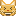## cumulative returns

dear sas members

hi

i'm trying to calculate cumulative returns, but i dont know how can i do...

i want to calculate monthly return by using daily returns.

[(1+R1)(1+R2)...(1+Rn)]-1

my data set:

date return(%)

19910801 5

19910802 3

19910803 2

19910804 3

...

19910831 3

19910901 -1

19910902 -0.8

...

(from 1991081 to 20151231)

Is there any way to calculate cumulative return for each month?

Thanks,

1 ACCEPTED SOLUTION

Accepted Solutions

## Re: cumulative returns

Instead of keeping running products, sum the logs of (1+returnpct/100).  At the end of the month take the antilog and subtract 1 (and mult by 100 if you want percentages).

In SAS, you can merge a dataset with itself, but offset by one record (see "firstobs=2") to determine whether the record in hand is the last for the current month:

``````data mreturns (drop=sumlog);
merge dreturns  dreturns(firstobs=2 keep=date rename=(date=nxtdate));
retain sumlog 0;
sumlog=sum(sumlog,log10(1+returnpct/100));
if month(date)^=month(nxtdate) then do;
mreturn=10**sumlog-1;
output;
sumlog=0;
end;
run;
``````
--------------------------
The hash OUTPUT method will overwrite a SAS data set, but not append. That can be costly. Consider voting for Add a HASH object method which would append a hash object to an existing SAS data set

Would enabling PROC SORT to simultaneously output multiple datasets be useful? Then vote for
Allow PROC SORT to output multiple datasets

--------------------------
3 REPLIES 3

## Re: cumulative returns

Hi,

Generally a good idea to search for things first, I have seen at least three similar posts in the last day or two:

https://communities.sas.com/t5/forums/searchpage/tab/message?q=cumulative

## Re: cumulative returns

Instead of keeping running products, sum the logs of (1+returnpct/100).  At the end of the month take the antilog and subtract 1 (and mult by 100 if you want percentages).

In SAS, you can merge a dataset with itself, but offset by one record (see "firstobs=2") to determine whether the record in hand is the last for the current month:

``````data mreturns (drop=sumlog);
merge dreturns  dreturns(firstobs=2 keep=date rename=(date=nxtdate));
retain sumlog 0;
sumlog=sum(sumlog,log10(1+returnpct/100));
if month(date)^=month(nxtdate) then do;
mreturn=10**sumlog-1;
output;
sumlog=0;
end;
run;
``````
--------------------------
The hash OUTPUT method will overwrite a SAS data set, but not append. That can be costly. Consider voting for Add a HASH object method which would append a hash object to an existing SAS data set

Would enabling PROC SORT to simultaneously output multiple datasets be useful? Then vote for
Allow PROC SORT to output multiple datasets

--------------------------

## Re: cumulative returns

i'm not good at mathmatics... so i can't exactly understand about the steps....I solved it.

Thank you so much!

Discussion stats
• 3 replies
• 740 views
• 1 like
• 3 in conversation